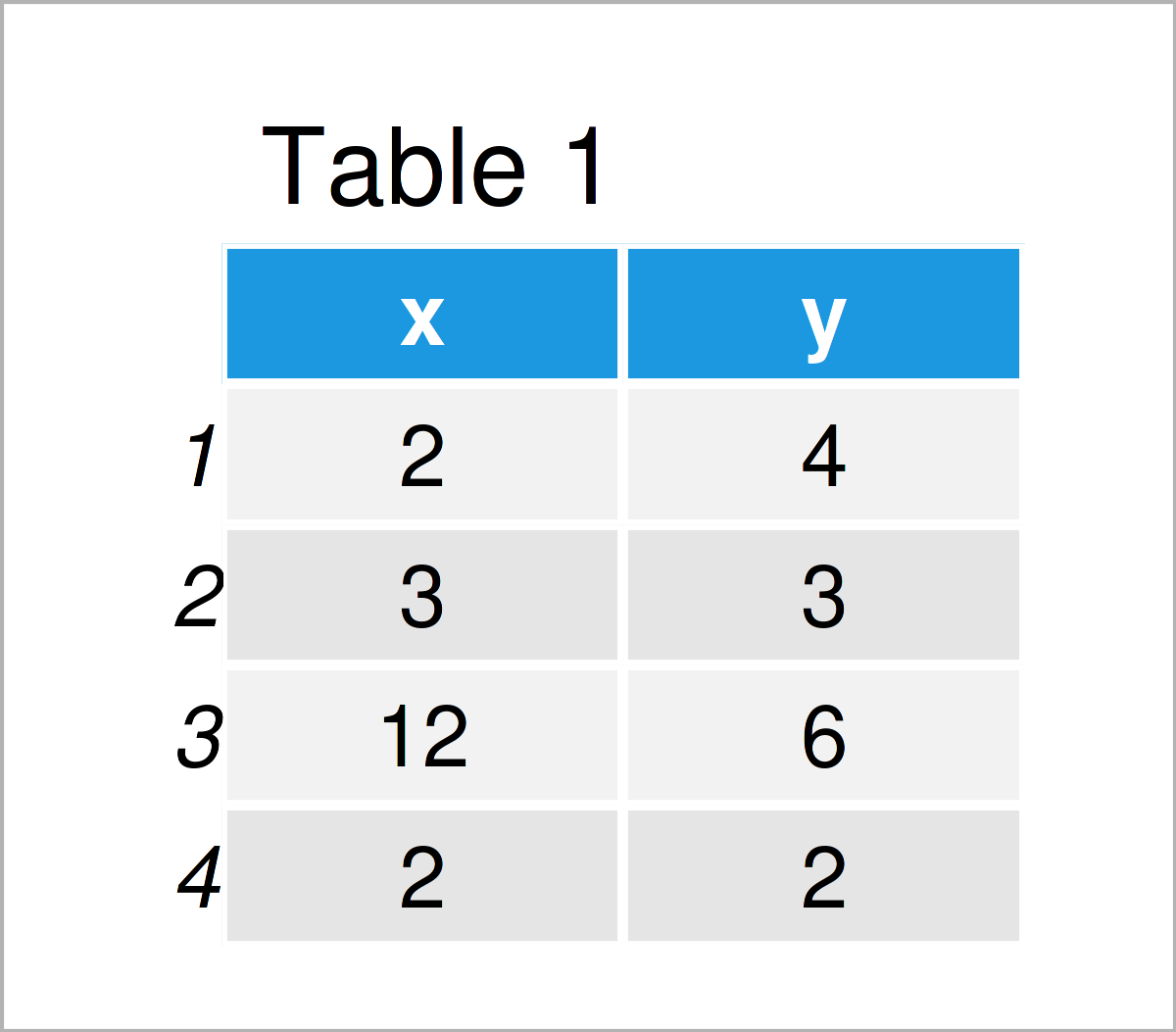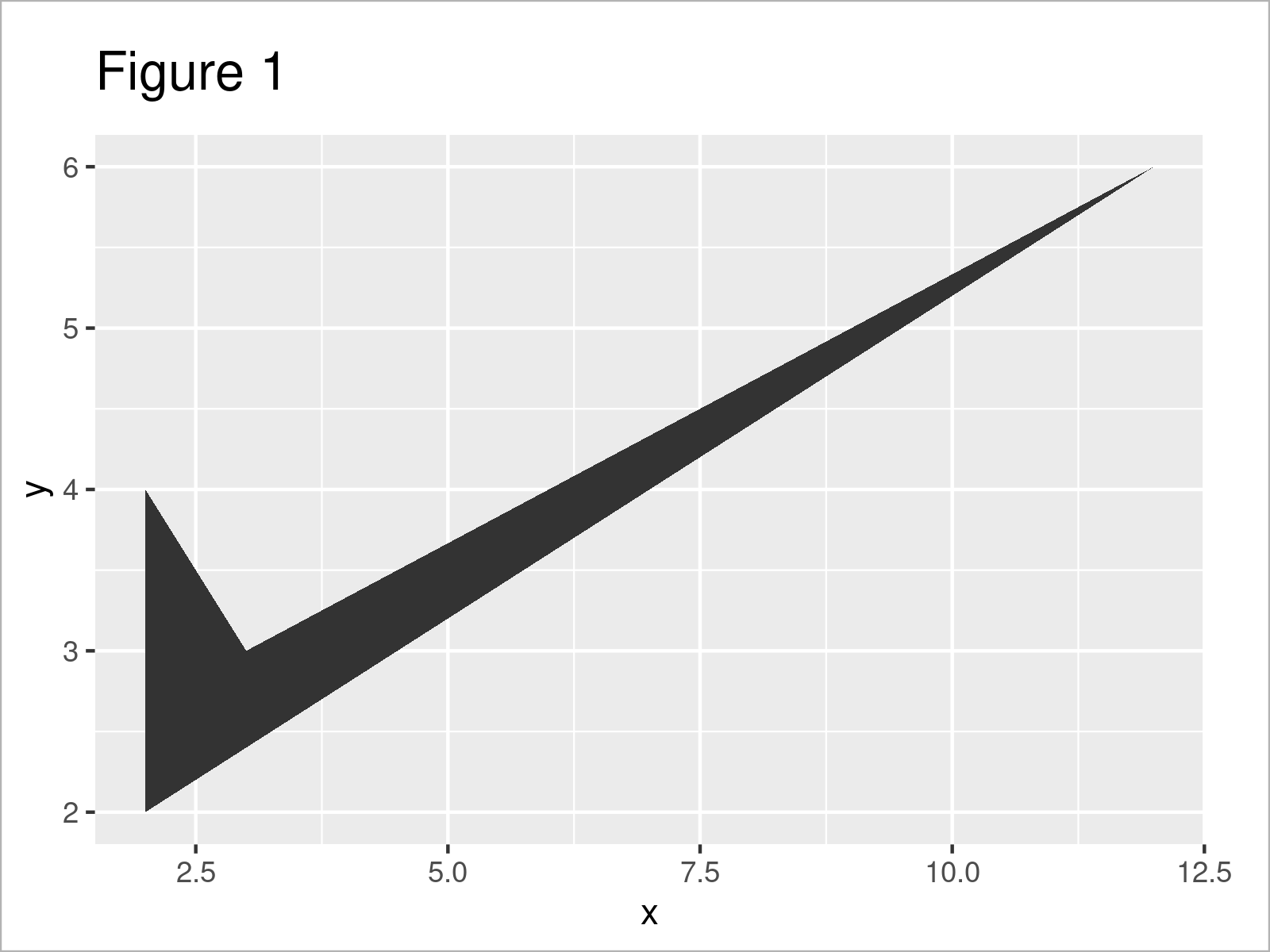# Remove Fill from ggplot2 Polygon Plot in R (Example)

In this R tutorial you’ll learn how to draw a ggplot2 polygon plot without filling color.

Let’s start right away…

## Exemplifying Data, Packages & Default Graphic

Let’s first create some example data:

```data <- data.frame(x = c(2, 3, 12, 2),    # Create example data frame
y = c(4, 3, 6, 2))
data                                      # Print example data frame```Table 1 illustrates the output of the RStudio console that got returned after executing the previous R code and shows that our example data contains four rows and two columns.

If we want to plot our data using the ggplot2 package, we also need to install and load ggplot2 to RStudio:

```install.packages("ggplot2")               # Install & load ggplot2
library("ggplot2")```

Next, we can draw our data using the geom_polygon function:

```ggplot(data, aes(x, y)) +                 # Draw polygon plot with default colors
geom_polygon()```The output of the previous code is illustrated in Figure 1 – A polygon plot with default fill.

## Example: Remove Filling Color from ggplot2 Polygon Graph

This example demonstrates how to avoid the filling color in a polygon plot created by the ggplot2 package.

To accomplish this, we have to specify the color argument within the geom_polygon function, and we have to set the fill argument to NA.

Let’s do this:

```ggplot(data, aes(x, y)) +                 # Draw polygon plot without filling
geom_polygon(color = "black",
fill = NA)```Figure 2 shows the output of the previous code: A ggplot2 polygon graph without fillings.

## Video, Further Resources & Summary

Would you like to know more about the creation of a ggplot2 polygon plot without filling color? Then you could watch the following video that I have published on my YouTube channel. I’m explaining the R programming codes of this article in the video.

Please accept YouTube cookies to play this video. By accepting you will be accessing content from YouTube, a service provided by an external third party.If you accept this notice, your choice will be saved and the page will refresh.

Furthermore, you could have a look at the other articles on my website.

In this article you have learned how to create a ggplot2 polygon plot without a filling color in the R programming language. Let me know in the comments below, if you have further questions.

Subscribe to the Statistics Globe Newsletter